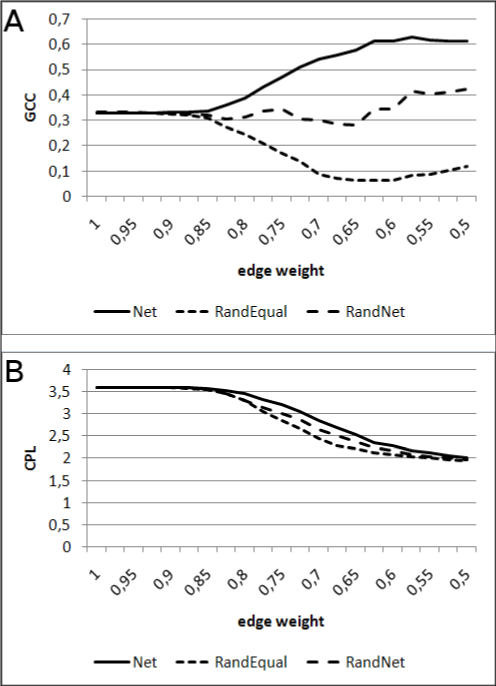Figure 5: Global clustering coefficient (GCC) (A) and characteristic path length (CPL) (B) in relation to edge weight computed for the edge weight interval [0.5,1.0]. GCC and CPL are provided for the INT1 graph (only including edges with experimental background) extended by inferred edges according to equation 5 (Net), by extending with the same number of edges being randomly distributed between nodes (RandEqual), and by the adding the same number of edges as for Net but in addition taking the graph degree distribution of the computed graph (Net) into account (RandNet).